Anúncio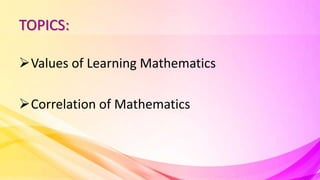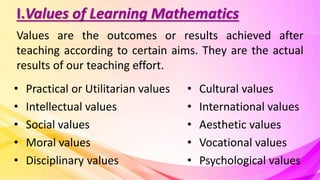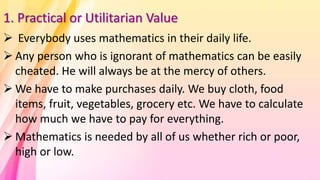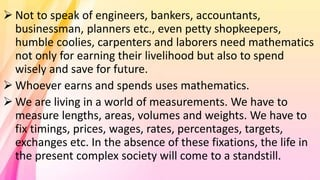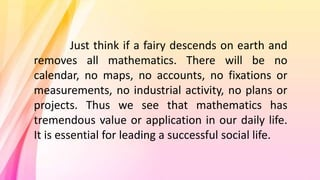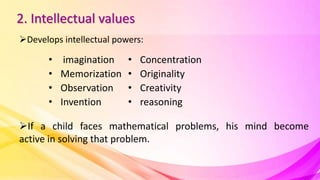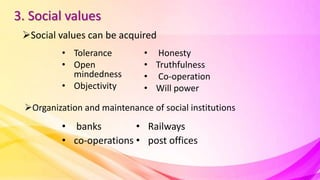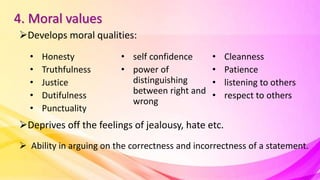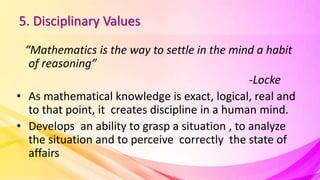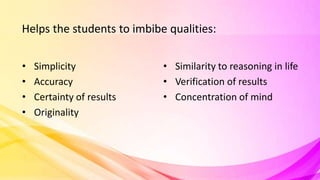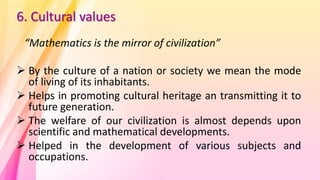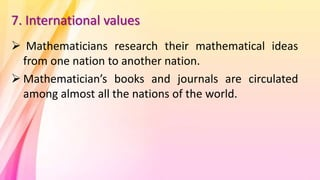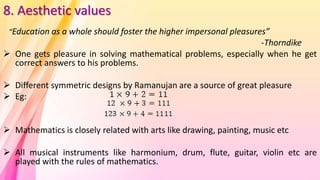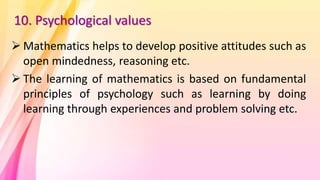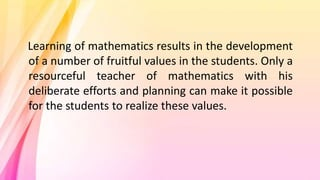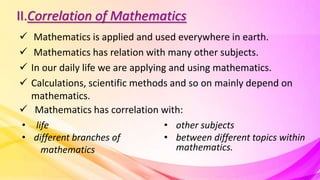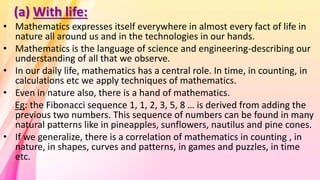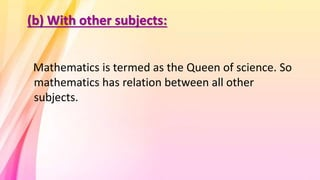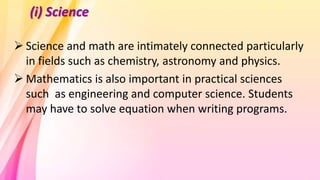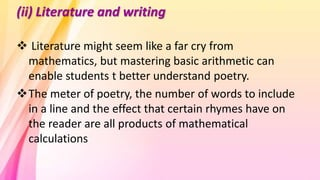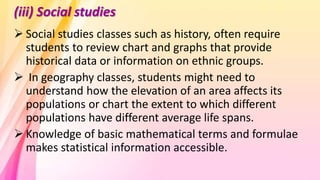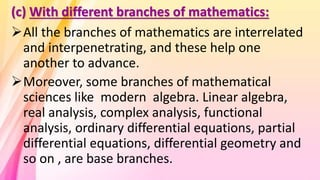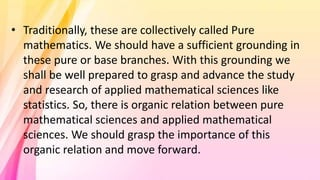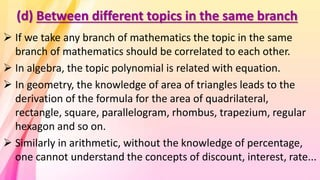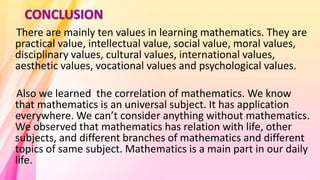1 de 29
Anúncio

### Values of learning mathematics & correlation of mathematics

1. TOPICS: Values of Learning Mathematics Correlation of Mathematics
2. I.Values of Learning Mathematics Values are the outcomes or results achieved after teaching according to certain aims. They are the actual results of our teaching effort. • Practical or Utilitarian values • Intellectual values • Social values • Moral values • Disciplinary values • Cultural values • International values • Aesthetic values • Vocational values • Psychological values
3. 1. Practical or Utilitarian Value  Everybody uses mathematics in their daily life.  Any person who is ignorant of mathematics can be easily cheated. He will always be at the mercy of others.  We have to make purchases daily. We buy cloth, food items, fruit, vegetables, grocery etc. We have to calculate how much we have to pay for everything.  Mathematics is needed by all of us whether rich or poor, high or low.
4.  Not to speak of engineers, bankers, accountants, businessman, planners etc., even petty shopkeepers, humble coolies, carpenters and laborers need mathematics not only for earning their livelihood but also to spend wisely and save for future.  Whoever earns and spends uses mathematics.  We are living in a world of measurements. We have to measure lengths, areas, volumes and weights. We have to fix timings, prices, wages, rates, percentages, targets, exchanges etc. In the absence of these fixations, the life in the present complex society will come to a standstill.
5. Just think if a fairy descends on earth and removes all mathematics. There will be no calendar, no maps, no accounts, no fixations or measurements, no industrial activity, no plans or projects. Thus we see that mathematics has tremendous value or application in our daily life. It is essential for leading a successful social life.
6. 2. Intellectual values Develops intellectual powers: • imagination • Memorization • Observation • Invention • Concentration • Originality • Creativity • reasoning If a child faces mathematical problems, his mind become active in solving that problem.
7. 3. Social values Social values can be acquired • Tolerance • Open mindedness • Objectivity • Honesty • Truthfulness • Co-operation • Will power Organization and maintenance of social institutions • banks • co-operations • Railways • post offices
8. 4. Moral values Develops moral qualities: • Honesty • Truthfulness • Justice • Dutifulness • Punctuality • self confidence • power of distinguishing between right and wrong • Cleanness • Patience • listening to others • respect to others Deprives off the feelings of jealousy, hate etc.  Ability in arguing on the correctness and incorrectness of a statement.
9. 5. Disciplinary Values “Mathematics is the way to settle in the mind a habit of reasoning” -Locke • As mathematical knowledge is exact, logical, real and to that point, it creates discipline in a human mind. • Develops an ability to grasp a situation , to analyze the situation and to perceive correctly the state of affairs
10. Helps the students to imbibe qualities: • Simplicity • Accuracy • Certainty of results • Originality • Similarity to reasoning in life • Verification of results • Concentration of mind
11. 6. Cultural values “Mathematics is the mirror of civilization”  By the culture of a nation or society we mean the mode of living of its inhabitants.  Helps in promoting cultural heritage an transmitting it to future generation.  The welfare of our civilization is almost depends upon scientific and mathematical developments.  Helped in the development of various subjects and occupations.
12. 7. International values  Mathematicians research their mathematical ideas from one nation to another nation.  Mathematician’s books and journals are circulated among almost all the nations of the world.
13. 8. Aesthetic values “Education as a whole should foster the higher impersonal pleasures” -Thorndike  One gets pleasure in solving mathematical problems, especially when he get correct answers to his problems.  Different symmetric designs by Ramanujan are a source of great pleasure  Eg:  Mathematics is closely related with arts like drawing, painting, music etc  All musical instruments like harmonium, drum, flute, guitar, violin etc are played with the rules of mathematics.
14. 9. Vocational values  Mathematics has great vocational values.  Study of mathematics prepares the students for a wide variety of vocations. It finds extensive application in all vocations like: • Agriculture • Accountancy • Banking • Business • Engineering • information technology • Tailoring • Carpentry • surveying etc.
15. 10. Psychological values  Mathematics helps to develop positive attitudes such as open mindedness, reasoning etc.  The learning of mathematics is based on fundamental principles of psychology such as learning by doing learning through experiences and problem solving etc.
16. Learning of mathematics results in the development of a number of fruitful values in the students. Only a resourceful teacher of mathematics with his deliberate efforts and planning can make it possible for the students to realize these values.
17. II.Correlation of Mathematics  Mathematics is applied and used everywhere in earth.  Mathematics has relation with many other subjects.  In our daily life we are applying and using mathematics.  Calculations, scientific methods and so on mainly depend on mathematics.  Mathematics has correlation with: • life • different branches of mathematics • other subjects • between different topics within mathematics.
18. (a) With life: • Mathematics expresses itself everywhere in almost every fact of life in nature all around us and in the technologies in our hands. • Mathematics is the language of science and engineering-describing our understanding of all that we observe. • In our daily life, mathematics has a central role. In time, in counting, in calculations etc we apply techniques of mathematics. • Even in nature also, there is a hand of mathematics. Eg: the Fibonacci sequence 1, 1, 2, 3, 5, 8 … is derived from adding the previous two numbers. This sequence of numbers can be found in many natural patterns like in pineapples, sunflowers, nautilus and pine cones. • If we generalize, there is a correlation of mathematics in counting , in nature, in shapes, curves and patterns, in games and puzzles, in time etc.
19. (b) With other subjects: Mathematics is termed as the Queen of science. So mathematics has relation between all other subjects.
20. (i) Science  Science and math are intimately connected particularly in fields such as chemistry, astronomy and physics.  Mathematics is also important in practical sciences such as engineering and computer science. Students may have to solve equation when writing programs.
21. (ii) Literature and writing  Literature might seem like a far cry from mathematics, but mastering basic arithmetic can enable students t better understand poetry. The meter of poetry, the number of words to include in a line and the effect that certain rhymes have on the reader are all products of mathematical calculations
22. (iii) Social studies  Social studies classes such as history, often require students to review chart and graphs that provide historical data or information on ethnic groups.  In geography classes, students might need to understand how the elevation of an area affects its populations or chart the extent to which different populations have different average life spans.  Knowledge of basic mathematical terms and formulae makes statistical information accessible.
23. (iv) The arts  Students interested in pursuing careers in theater, music, dance or art can be benefit from basic mathematical knowledge.  Musical rhythm often follows complex mathematical series, and math can help students learn the basic rhythms of dances used in bullet and theater performances.  Art thrives an geometry, and students who understand basic geometric formulae can craft impressive art pieces.
24. (c) With different branches of mathematics: All the branches of mathematics are interrelated and interpenetrating, and these help one another to advance. Moreover, some branches of mathematical sciences like modern algebra. Linear algebra, real analysis, complex analysis, functional analysis, ordinary differential equations, partial differential equations, differential geometry and so on , are base branches.
25. • Traditionally, these are collectively called Pure mathematics. We should have a sufficient grounding in these pure or base branches. With this grounding we shall be well prepared to grasp and advance the study and research of applied mathematical sciences like statistics. So, there is organic relation between pure mathematical sciences and applied mathematical sciences. We should grasp the importance of this organic relation and move forward.
26. (d) Between different topics in the same branch  If we take any branch of mathematics the topic in the same branch of mathematics should be correlated to each other.  In algebra, the topic polynomial is related with equation.  In geometry, the knowledge of area of triangles leads to the derivation of the formula for the area of quadrilateral, rectangle, square, parallelogram, rhombus, trapezium, regular hexagon and so on.  Similarly in arithmetic, without the knowledge of percentage, one cannot understand the concepts of discount, interest, rate...
27. CONCLUSION There are mainly ten values in learning mathematics. They are practical value, intellectual value, social value, moral values, disciplinary values, cultural values, international values, aesthetic values, vocational values and psychological values. Also we learned the correlation of mathematics. We know that mathematics is an universal subject. It has application everywhere. We can’t consider anything without mathematics. We observed that mathematics has relation with life, other subjects, and different branches of mathematics and different topics of same subject. Mathematics is a main part in our daily life.
28. THANK YOU
Anúncio